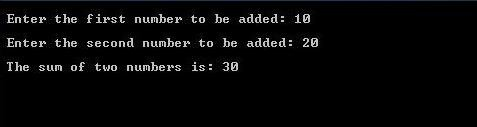# C PROGRAM TO ADD TWO NUMBERS / INTEGERS

In this post, we will see a C Program to read two number inputs from user and display the sum.

The program below accepts two numbers from user and perform basic arithmetic operation(addition) using `+` operator and display the sum on screen.

For algorithm & flowchart to add two numbers, go through the post given below.

### Program :

Sample Output :Output Text :
Enter the first number to be added: 10
Enter the second number to be added: 20
The sum of two numbers is: 30

### Explanation for the above program :

1) The #include<stdio.h> includes/loads the file stdio.h into your program while execution. so program can access the in-built functions such as printf(), scanf() etc.

2) main() is the main function where the program execution starts. int is the default return type of main function.

3) The int is a keyword used to declare a variable of type integer(i.e, its allows only a whole number, not decimal/float ). i.e. num1, num2, sum are variables of type int.

4) Printf() is a function that prints the text on output screen. And \n is a New Line escape sequence character used to print new line in output.

5) scanf() is a function used to accept input from keyboard.

scanf(“%d”,&num1); reads for the input from the keyboard and store the value in num1.

6) sum = num1 + num2;  the sum of the values of num1 and num2 is stored in the variable sum.

7) return(0); tells the OS that the program ended successfully.